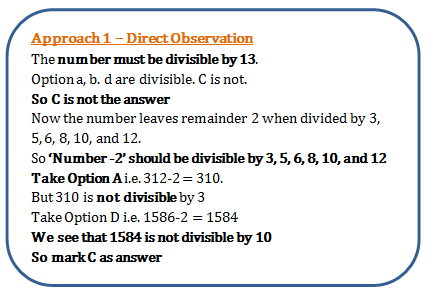# Problems on H.C.F and L.C.M - Quantitative Aptitude (MCQ) questions for Q. 29228

Q.  What is the least number which when divided by the numbers 3, 5, 6, 8, 10 and 12 leaves in each case a remainder 2 but which when divided by 13 leaves no remainder?
- Published on 07 Jul 17

a. 312
b. 962
c. 1586
d. 1562#### Discussion

• Tushar Paul   -Posted on 11 Oct 21
Option a,b and c divisible but d is not ...and answer would be c.

## ➨ Post your comment / Share knowledgeEnter the code shown above:

(Note: If you cannot read the numbers in the above image, reload the page to generate a new one.)